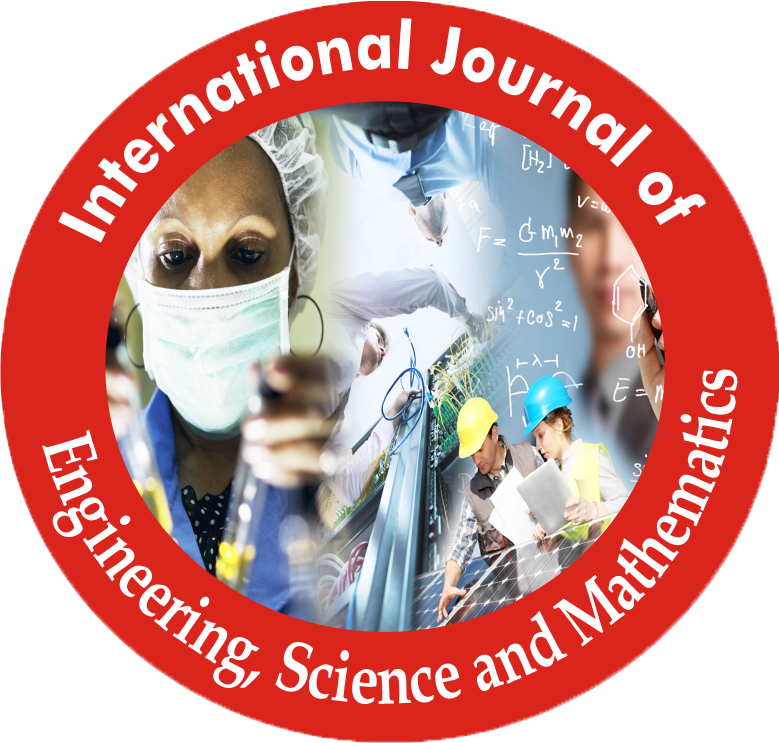# International Journal of Engineering, Science and Mathematics(IJMRA Publications)-Peer Reviewed Refereed JournalPages: 269-273

Date of Publication: 17-Apr-2018

## ALTERNATIVE SAMPLING STRATEGY BASED UPON COEFFICIENT OF VARIATION WHEN AUXILIARY INFORMATION IS AVAILABLE

Author: Dr. Spersh Bhatt*

Category: Engineering, Science and Mathematics

Abstract:

It is well know that in order to improve the efficiencies of the estimates probability sampling is preferred over non probability sampling. If the difference in the size of the units is large enough to affect the study, we make use of PPS sampling where the probability of selecting a unit is proportional to the size measure of the unit. Sometimes we may be confronted with situations where information on a character closely related to the main variable is available from a previous study or other secondary sources. Various authors have utilized this auxiliary information by taking the initial probability of selection equal to the size measure of the auxiliary information. This scheme however fails to give best results when the population under consideration is skewed. The paper presents an alternative without replacement sampling strategy obtained by utilizing auxiliary information to modify the initial probability of selection of the units. A computer program was developed in Visual Basic to find out the probabilities of selection and the variance of the sampling strategy proposed using the Horvitz Thompson estimator of population total. The empirical comparison of the proposed strategy with the existing Midzuno-Sen strategy shows that the proposed scheme performs better than the Midzuno-Sen strategy when the population is skewed.

Keywords: Coefficient Of Variation, Probability Of Selection, Relative Efficiency, Sampling Strategy, Sampling Design